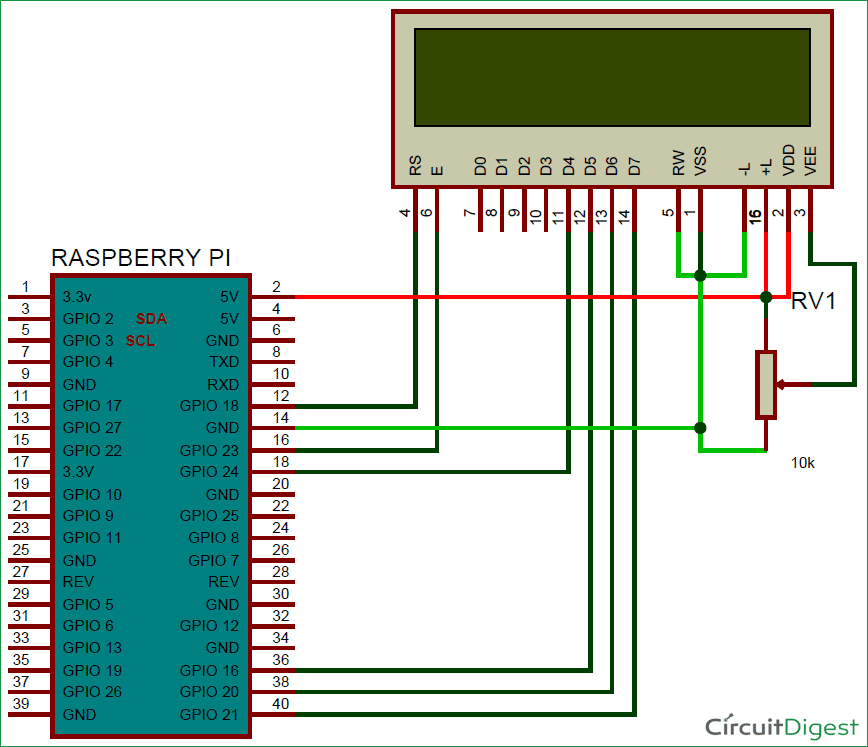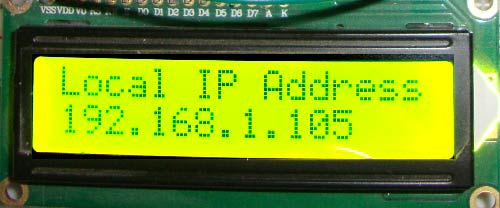The main problem while working with Raspberry Pi is to know the IP address of the Raspberry Pi, which will be needed to login into it using some SSH or file transfer client. So today we will share some Python scripts to find the local IP address of your Raspberry Pi on the network and display it on the 16x2 LCD Screen. We will also add the script in the Crontab so that it can be run on every 10 minutes and we will have the updated IP address every time.

### Interfacing 16x2 LCD with Raspberry Pi:

Before we will find the IP address of the Raspberry PI, first we need to interface 16x2 LCD with Raspberry Pi. Here in this Project we have used an external Adafruit Library for interfacing the 16x2 LCD with Raspberry Pi, using which you don’t need to write many lines of code to drive the LCD and you can directly print on LCD by just using one line of code. However this Library is created by Adafruit but it can used for any LCD module which has HD44780 controller.

To use the Adafruit Library, we first need to install it by using below commands. First command will clone the CharLCD repository (by Adafruit) on your Raspberry Pi, second command will  take you inside that downloaded directory and finally we need to execute setup.py script, presented inside the Adafruit_Python_CharLCD directory, to install the library.

```git clone https://github.com/adafruit/Adafruit_Python_CharLCD.git
sudo python setup.py install```

Now the library for 16x2 LCD has been installed and you can use its functions by just importing this library in your python program using the below line:

`import Adafruit_CharLCD as LCD`

There are some example scripts inside the ‘examples’ folder which is present in the library folder (Adafruit_Python_CharLCD). You can test the setup by running char_lcd.py example script. But before that, you need to connect the LCD pins with the Raspberry Pi as given below in the circuit diagram in next section.

You can also connect LCD with some other GPIO pins of Raspberry Pi, all you need to mention the correct interfacing pins in your python program like below. Learn more about Raspberry Pi GPIO Pins here.

```# Raspberry Pi pin setup
lcd_rs = 18
lcd_en = 23
lcd_d4 = 24
lcd_d5 = 16
lcd_d6 = 20
lcd_d7 = 21
lcd_backlight = 2```

Now you can directly use the functions provided by Adafruit Library to control the LCD. Some of the functions are given below; you can find more in example script:

• lcd.message(message) = To print the text on LCD.
• lcd.clear() = To clear the LCD.
• set_cursor(col, row) = Move the cursor to any position at column and row.
• lcd.move_left() = To move the cursor to Left by one position.
• lcd.move_right() = To move the cursor to Right by one position.

If you want to connect the LCD without using any external library then you can check our previous tutorial, where we have written all the functions for 16x2 LCD. Check this one to interface the LCD in 8-bit Mode and this one to interface the LCD in 4-bit mode.

### Circuit Diagram:### Display IP Address of Raspberry Pi on LCD:

After interfacing 16x2 LCD with Raspberry Pi, now we need to get the IP address of Raspberry Pi and print it on LCD using Python Script. There are lot of ways to get the local IP address of Raspberry Pi, here we are describing three Python Scripts to get the IP address, you can use any of them.

Using Linux Commands:

On terminal, we can easily get the IP address by using hostname –I command, so if we can run the linux command from the python then we can get the IP address. So to run Linux commands from Python we need to import a library named commands, so the complete program will be like below:

```import time
import commands

# Raspberry Pi pin setup
lcd_rs = 18
lcd_en = 23
lcd_d4 = 24
lcd_d5 = 16
lcd_d6 = 20
lcd_d7 = 21
lcd_backlight = 2

# Define LCD column and row size for 16x2 LCD.
lcd_columns = 16
lcd_rows = 2

lcd = LCD.Adafruit_CharLCD(lcd_rs, lcd_en, lcd_d4, lcd_d5, lcd_d6, lcd_d7, lcd_columns, lcd_rows, lcd_backlight)
lcd.message(commands.getoutput('hostname -I'))

time.sleep(10.0)  # Wait 5 seconds
lcd.clear()```

You can also replace the hostname –I command in the program by the below command if you want to get the IP address by using more reliable ifconfig command:

lcd.message(commands.getoutput('ifconfig wlan0 | grep "inet\ addr" | cut -d: -f2 | cut -d" " -f1'))

Note: Repalce ‘wlan0’ with ‘eth0’ if your Raspberry Pi is on the Ethernet.

Using Socket programming:

Here in this program we will create a socket of family AF_INET and type SOCK_DGRAM using this line: s = socket.socket(socket.AF_INET, socket.SOCK_DGRAM), then we will create connection through the socket using some hostname or ip address like 8.8.8.8, you can also use some other website to connect with the socket like gmail.com. So we can get the local IP address of RPi from the socket which has been created for communication between Raspberry Pi and dummy IP address. Below is the full Program:

```import time
import socket

# Raspberry Pi pin setup
lcd_rs = 18
lcd_en = 23
lcd_d4 = 24
lcd_d5 = 16
lcd_d6 = 20
lcd_d7 = 21
lcd_backlight = 2

# Define LCD column and row size for 16x2 LCD.
lcd_columns = 16
lcd_rows = 2

lcd = LCD.Adafruit_CharLCD(lcd_rs, lcd_en, lcd_d4, lcd_d5, lcd_d6, lcd_d7, lcd_columns, lcd_rows, lcd_backlight)

s = socket.socket(socket.AF_INET, socket.SOCK_DGRAM)
s.connect(("8.8.8.8",80))
s.close()

# Wait 5 seconds
time.sleep(10.0)
lcd.clear()```

Note: Using “socket.gethostbyname(socket.gethostname())” will always give you Localhost IP address (127.0.0.1)

Using ‘fcntl’ Module:

This module performs file control and I/O control on file descriptors. Here it is used to extract the IP address from the network files. Below is the full Python code:

```import time
import socket
import fcntl
import struct

# Raspberry Pi pin setup
lcd_rs = 18
lcd_en = 23
lcd_d4 = 24
lcd_d5 = 16
lcd_d6 = 20
lcd_d7 = 21
lcd_backlight = 2

# Define LCD column and row size for 16x2 LCD.
lcd_columns = 16
lcd_rows = 2

lcd = LCD.Adafruit_CharLCD(lcd_rs, lcd_en, lcd_d4, lcd_d5, lcd_d6, lcd_d7, lcd_columns, lcd_rows, lcd_backlight)

s = socket.socket(socket.AF_INET, socket.SOCK_DGRAM)
return socket.inet_ntoa(fcntl.ioctl(
s.fileno(),
struct.pack('256s', network[:15])
)[20:24])

# Wait 5 seconds
time.sleep(10.0)
lcd.clear()```

Note: Repalce ‘wlan0’ with ‘eth0’ if your Raspberry Pi is on the Ethernet.### Execute Script Periodically using ‘crontab’:

Final step is to add the entry for running this script periodically on every 15 minutes so that we can get updated IP every time. To do this we need to edit the cron file using below command:

`crontab –e`

And then enter the below line at the bottom of the cron file and save it using CTRL + X, then Y, then enter.

`*/15 * * * * sudo python /home/pi/ip_address_lcd.py`

You can change the address according to location of your Python Script file and you can also change the duration in which you want repeatedly run the script to get the updated IP.

•